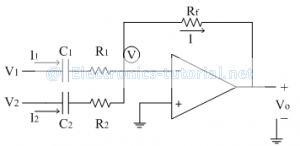Home > analog integrated circuits > opampdifferentiator > summing differentiator

Summing differentiator

The following figure shows the configuration of op-amp as summing differentiator.Let assume that the inverting terminal of op-amp is at potential 'V'
Due to virtual ground concept V= 0
Applying KCL at node 'V', we get
I=I1+I2Thus the above circuit gives addition of derivatives of two input signals V1 and V2. Thus acts as summing differentiator.